### Dual Variables, Reduced Costs, and Status

During optimization, dual variables and reduced costs are used to determine whether an arc, constraint slack, surplus, or nonarc variable should have a flow or value change. The ARCOUT= and CONOUT= data sets each have a variable called _RCOST_ that contains reduced cost values. In the CONOUT= data set, this variable also has the reduced costs of nonarc variables. For an arc, the reduced cost is the amount that would be added to the total cost if that arc were made to convey one more unit of flow. For a nonarc variable, the reduced cost is the amount that would be added to the total cost if the value currently assigned to that nonarc variable were increased by one.

During the optimization of a minimization problem, if an arc has a positive reduced cost, PROC NETFLOW takes steps to decrease the flow through it. If an arc has a negative reduced cost, PROC NETFLOW takes steps to increase the flow through it. At optimality, the reduced costs of arcs with flow at their respective lower bounds are nonnegative; otherwise, the optimizer would have tried to increase the flow, thereby decreasing the total cost. The _STATUS_ of each such nonbasic arc is LOWERBD NONBASIC. The reduced costs of arcs with flow at capacity are nonpositive. The _STATUS_ of each such nonbasic arc is UPPERBD NONBASIC. Even though it would decrease total cost, the optimizer cannot increase the flows through such arcs because of the capacity bound. Similar arguments apply for nonarc variables.

The reduced cost is also the amount that would be subtracted from the total cost if that arc was made to convey one less unit of flow. Similarly, a reduced cost is the amount subtracted from the total cost if the value currently assigned to that nonarc variable is decreased by one.

The dual variables and reduced costs can be used to detect whether multiple optimal solutions exist. A zero reduced cost of a nonbasic arc indicates the existence of multiple optimal solutions. A zero reduced cost indicates, by definition, that the flow through such arcs can be changed with zero change to the total cost. (Basic arcs and basic nonarc variables technically have zero reduced costs. A missing value is used for these so that reduced costs of nonbasic arcs and nonbasic nonarc variables that are zero are highlighted.)

The range over which costs can vary before the present solution becomes nonoptimal can be determined through examination of the reduced costs. For any nonbasic arc with assigned flow equal to its lower bound, the amount by which the cost must be decreased before it becomes profitable for this arc to convey additional flow is the value of its reduced cost. The cost reduction necessary for a nonbasic arc currently assigned capacity flow to undergo a worthwhile flow decrease is the absolute value of its reduced cost. In both cases, this minimum cost reduction changes the reduced cost to zero. Any further reduction promotes a possible basis change.

The reduced cost of an arc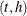is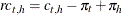where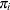is the dual value for nodeand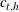is the cost of the arc with tail nodeand head node.

If the problem has side constraints and archas nonzero lhs coefficients, then the following term must be subtracted from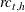: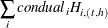where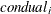is the dual variable of constraint, and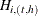is the coefficient of arcin constraint.

If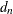is the objective function coefficient of nonarc variable, the reduced cost is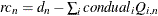, where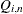is the coefficient of nonarc variablein constraint.